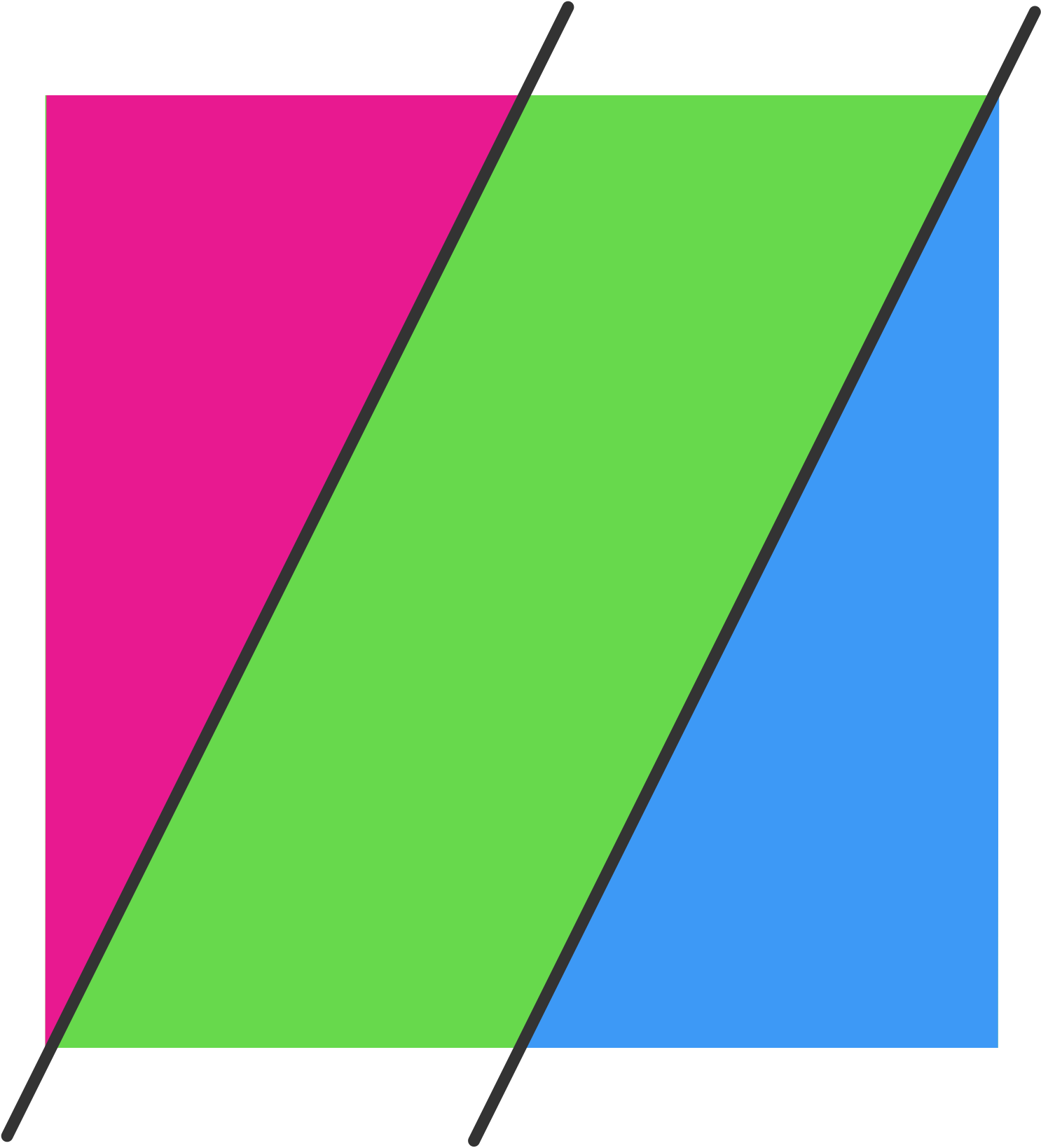# A cut above .... and below

Geometry Level 3Suppose a square is cut by two parallel lines at perpendicular distance $6$ cm. apart such that

(i) one line has an endpoint at the lower left corner of the square and the other has an endpoint at the upper right corner of the square, and

(ii) the square is divided into three regions, (a parallelogram and two triangles), of equal area.

What is the area of the square in cm$^{2}$?

×

Problem Loading...

Note Loading...

Set Loading...